# Frank Solutions for Class 9 Maths Chapter 17 Pythagoras Theorem

Frank Solutions Class 9 Maths Chapter 17 Pythagoras Theorem provides detailed answers for each and every question of the textbook. These solutions enable students to grasp the techniques in solving difficult problems effortlessly. Frank Solutions helps in boosting revision among students and appear for the examination without fear. Various problems are available in this resource to help students understand the skills of solving problems within a short duration. For more effective learning, students are suggested to download Frank Solutions Class 9 Maths Chapter 17 Pythagoras Theorem PDF, from the links given here

Chapter 17 of Frank Solutions Class 9 provides information about Pythagoras Theorem. This theorem states that, in a right angled triangle, the square of the hypotenuse is equal to the sum of the squares of the other two sides. These solutions are the best study source for ICSE students as the answers help them to clear their doubts quickly, along with making them curious to study further.

## Frank Solutions for Class 9 Maths Chapter 17 Pythagoras Theorem Download PDF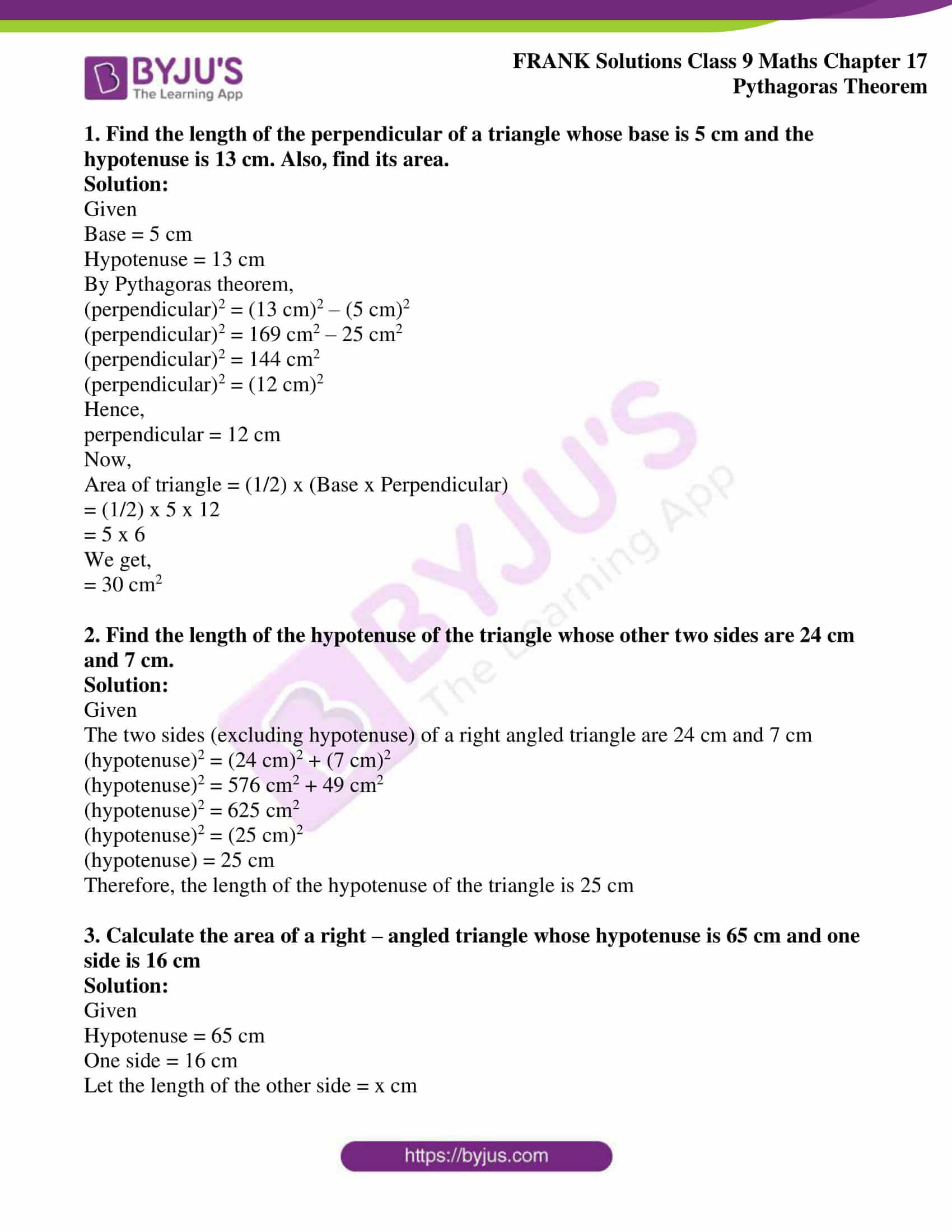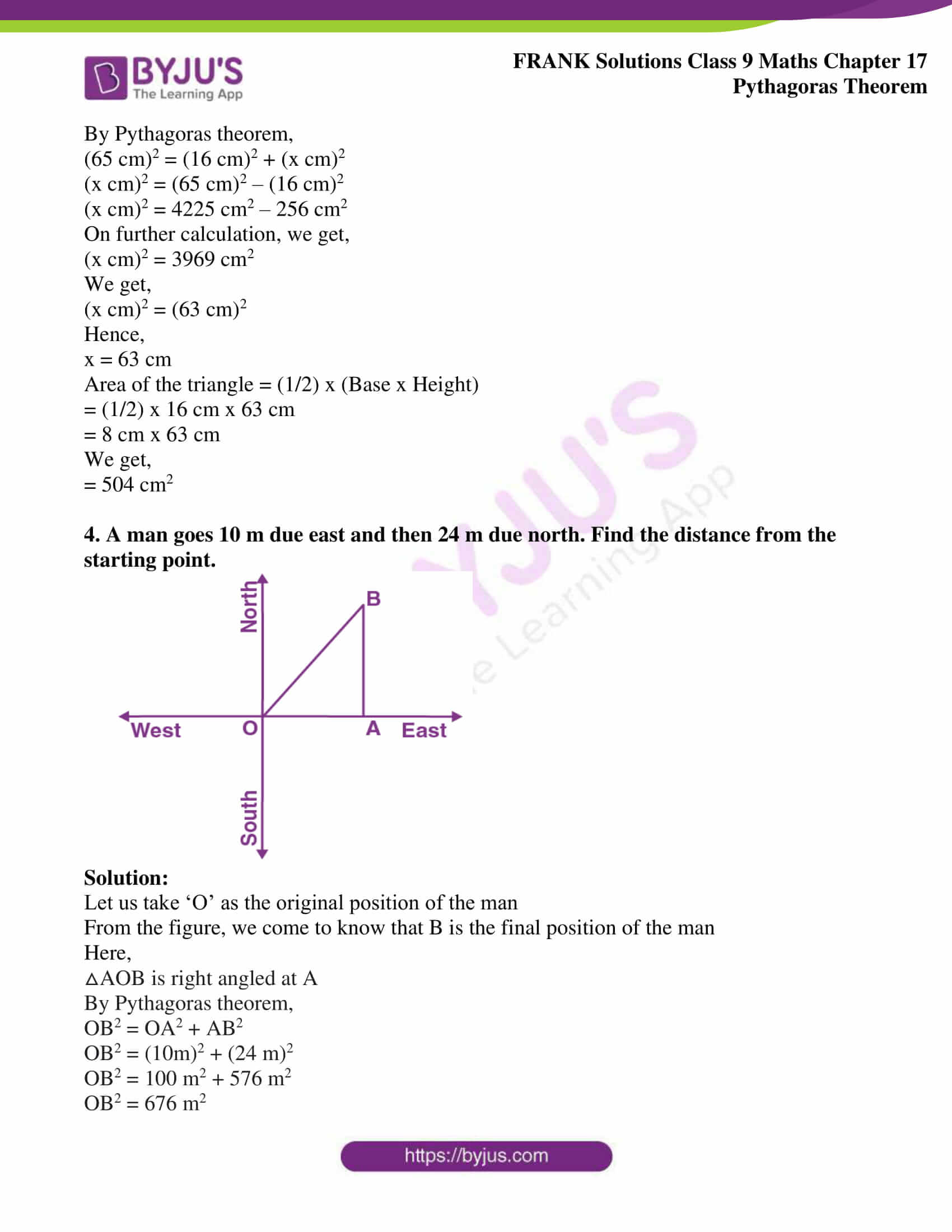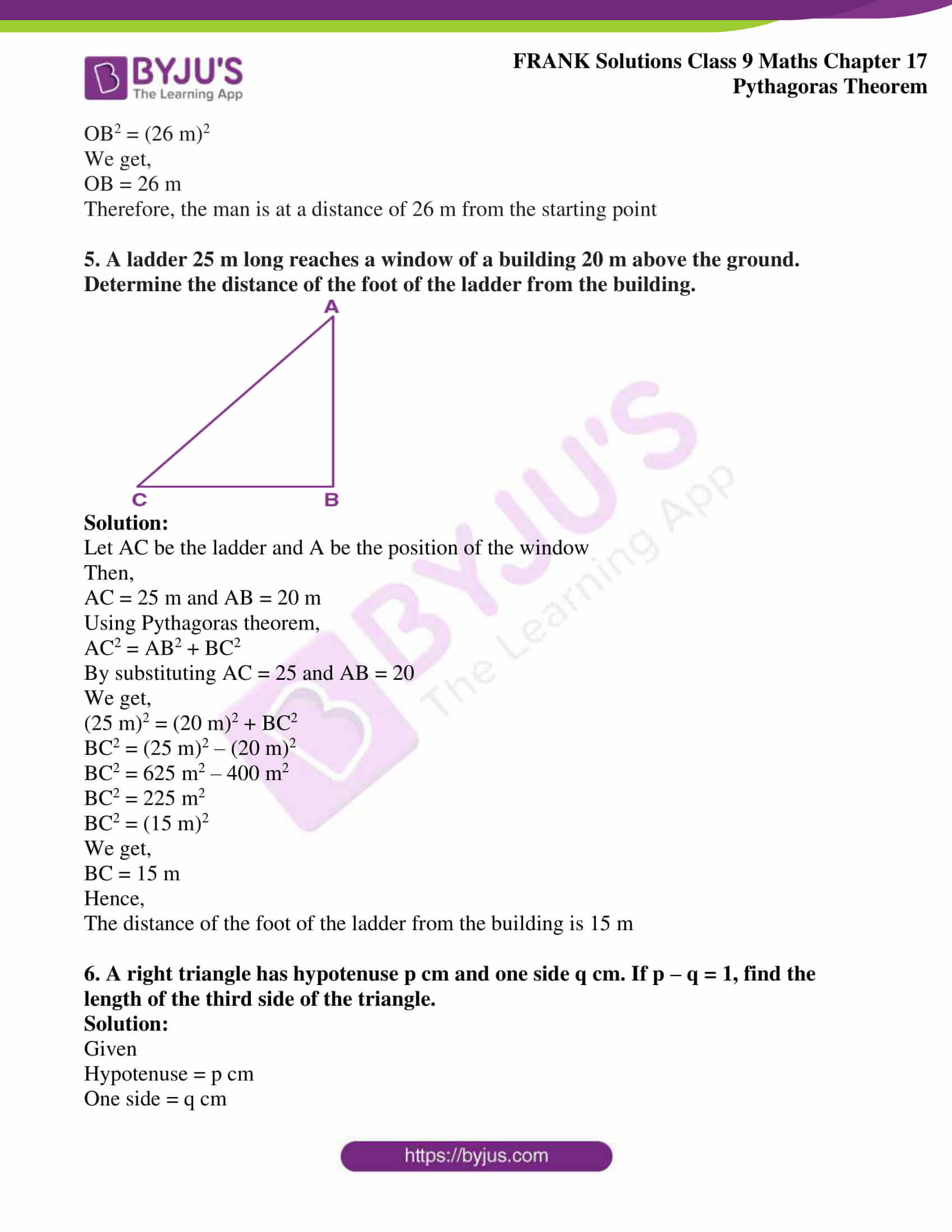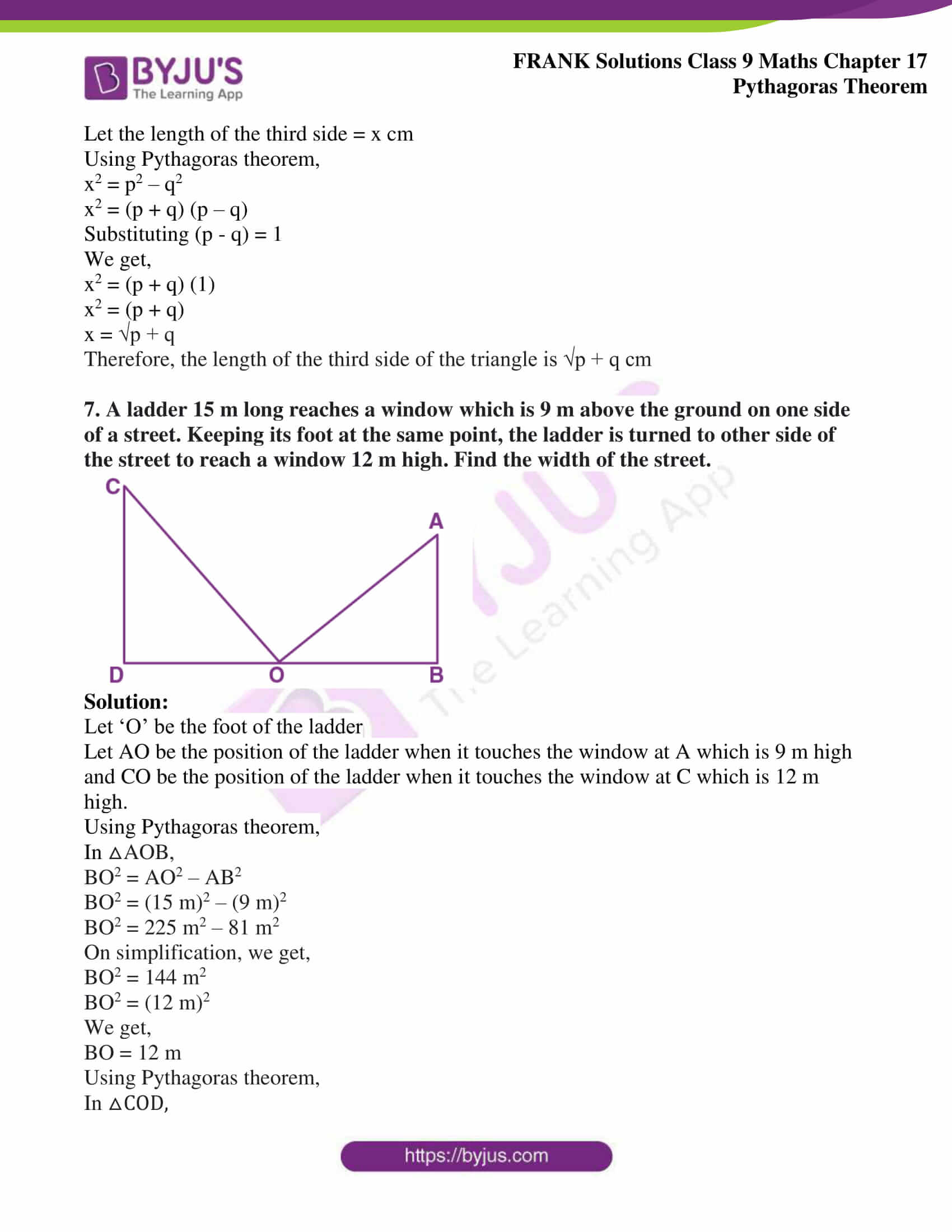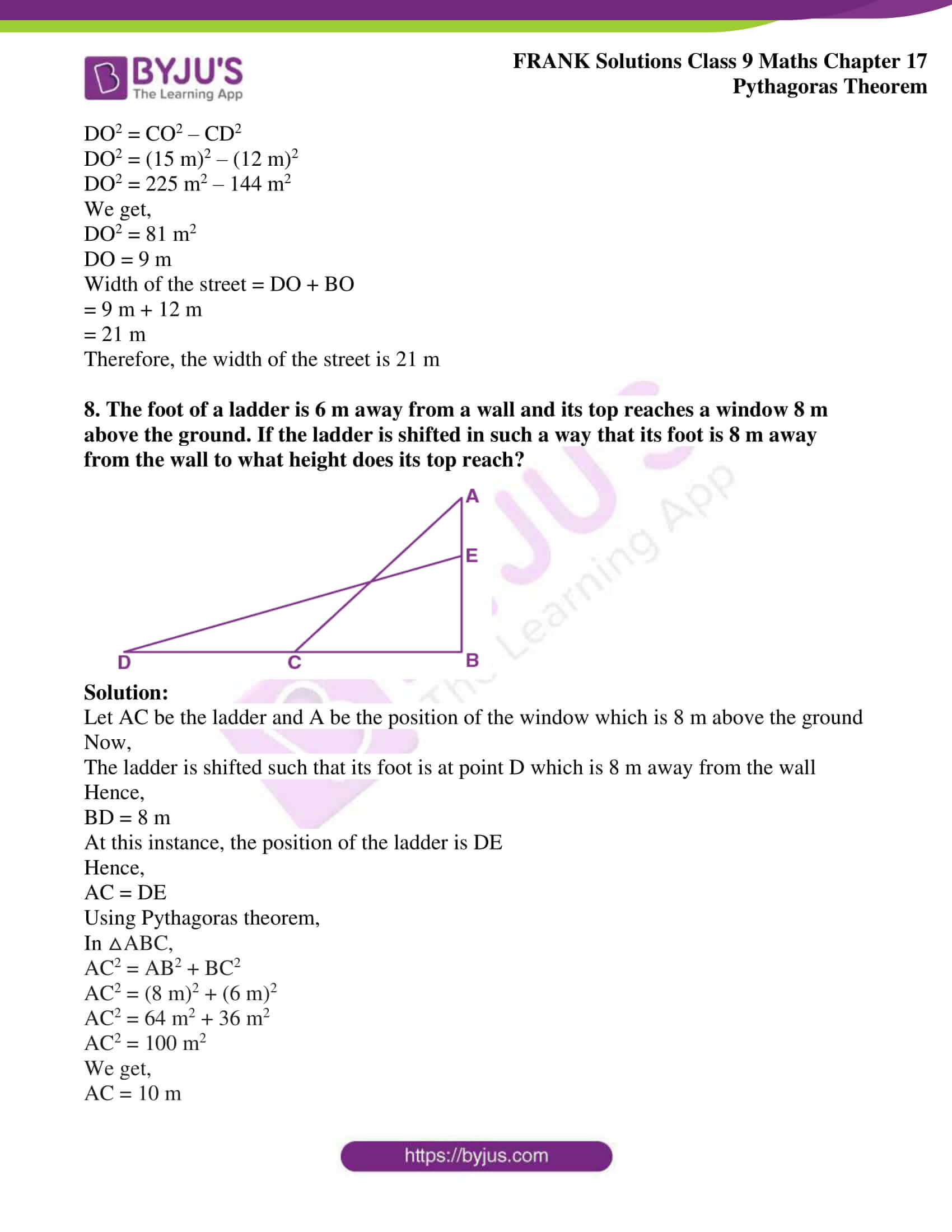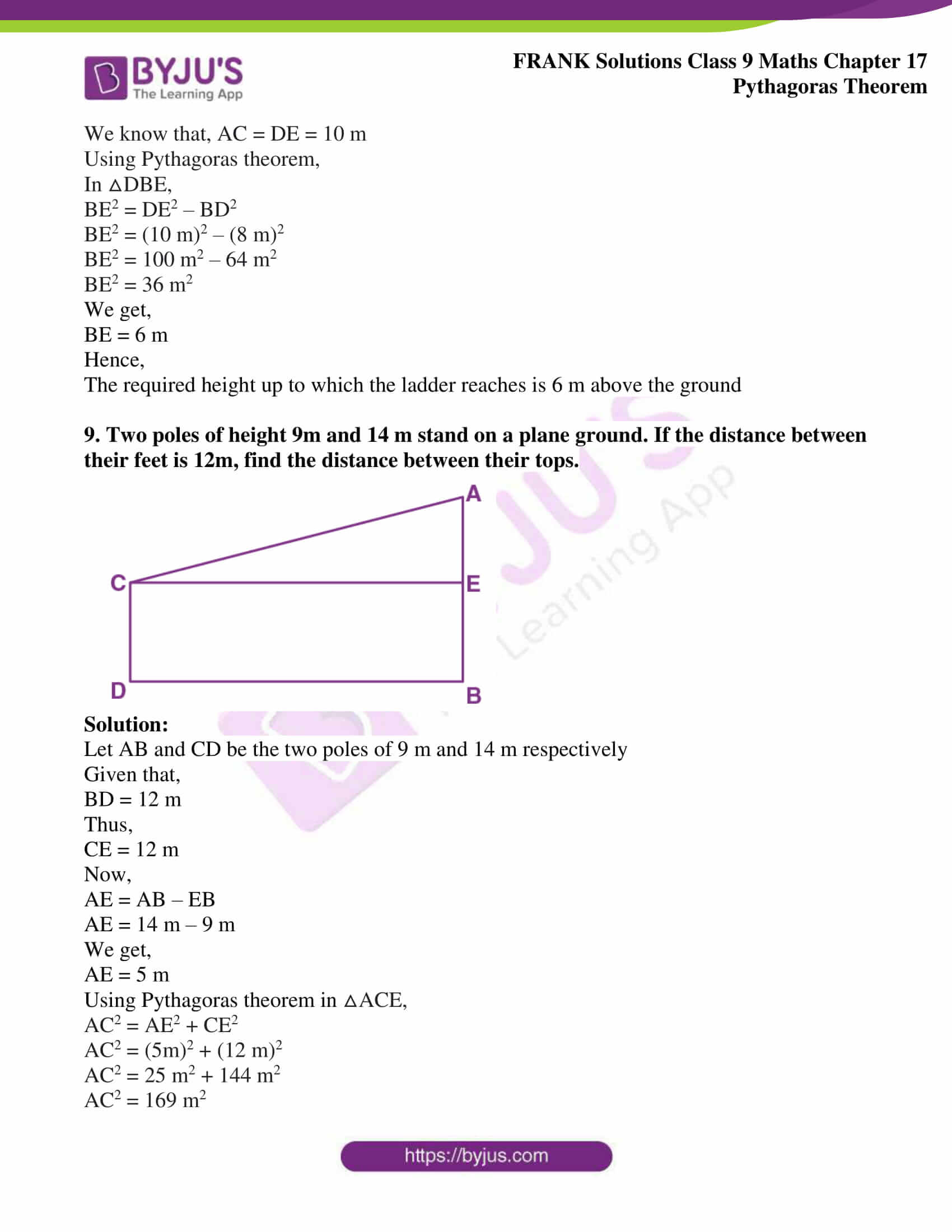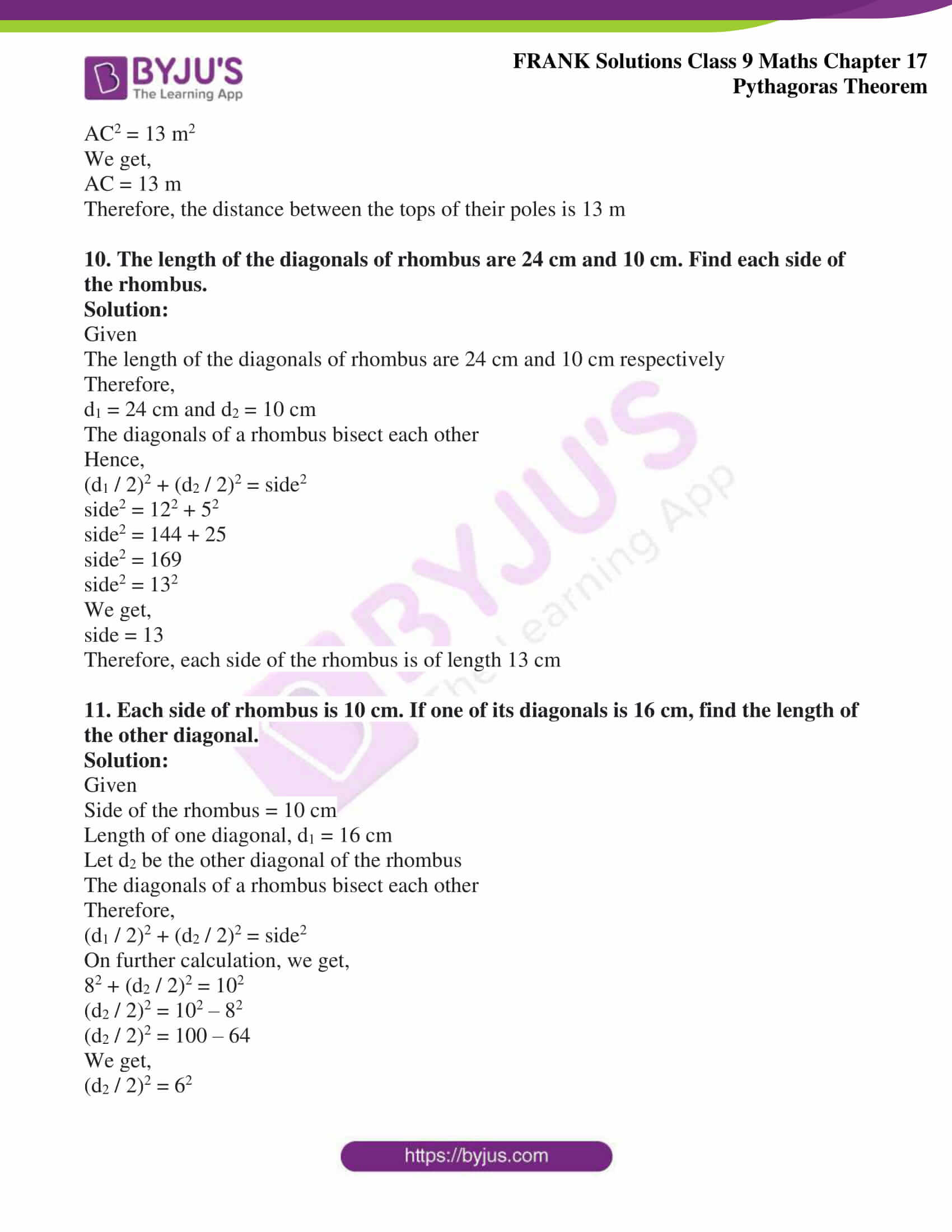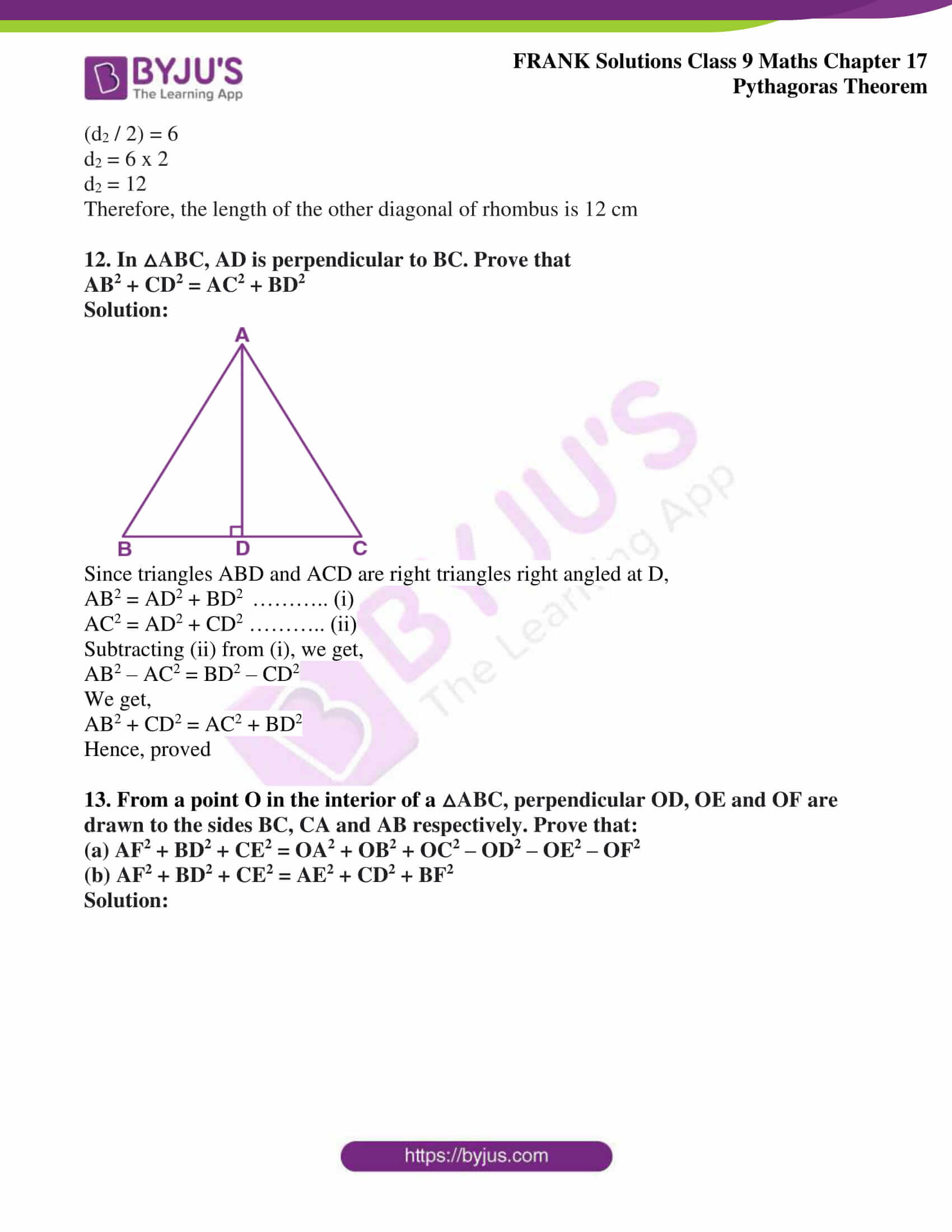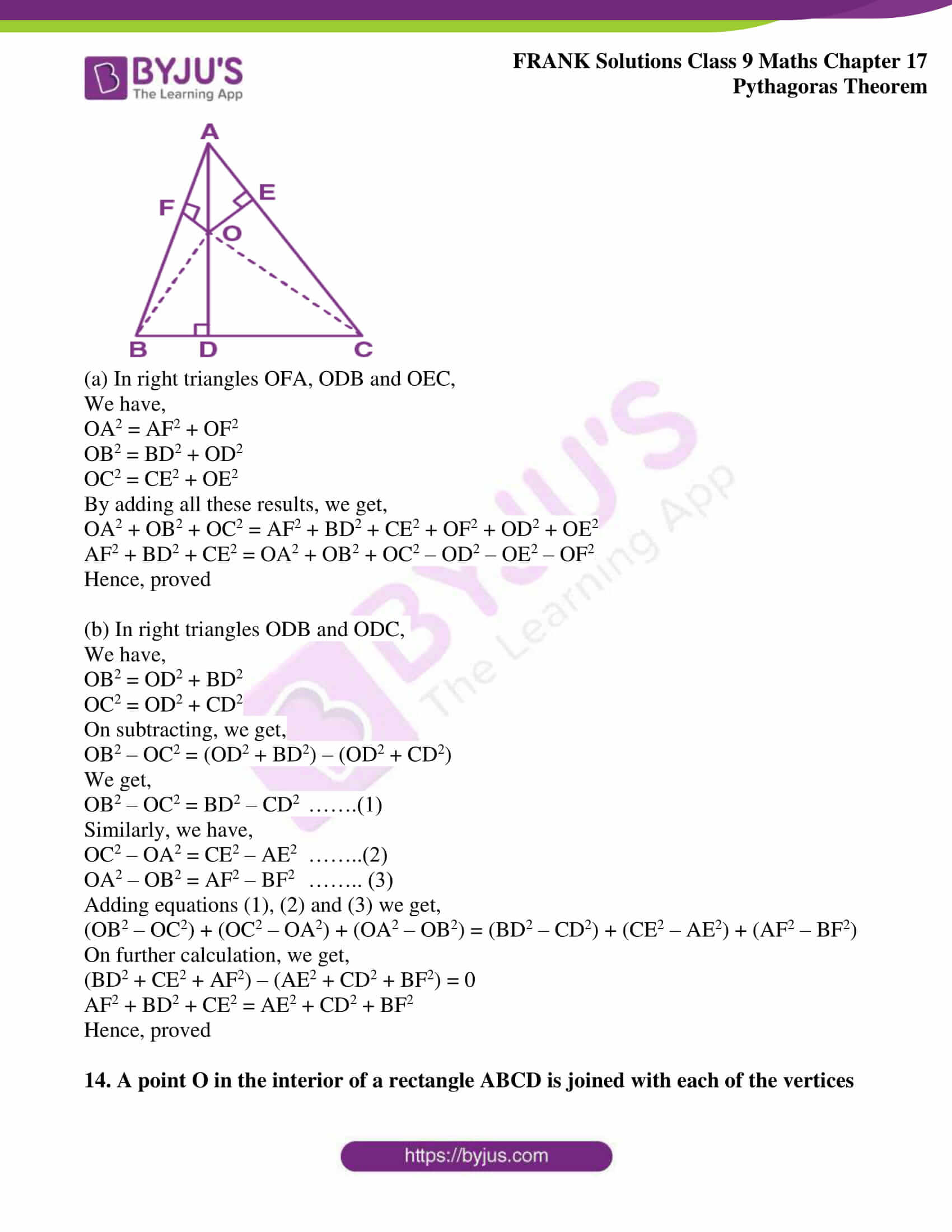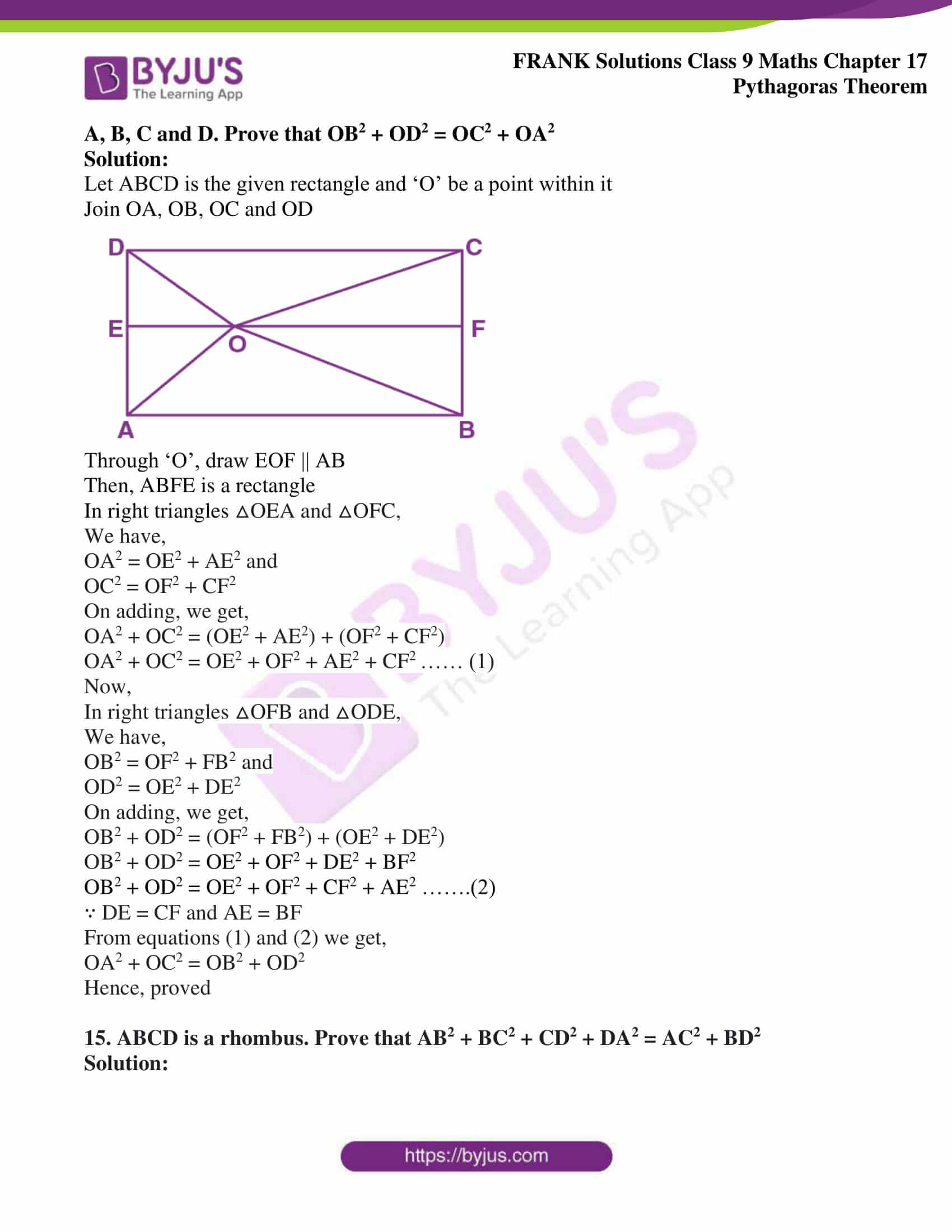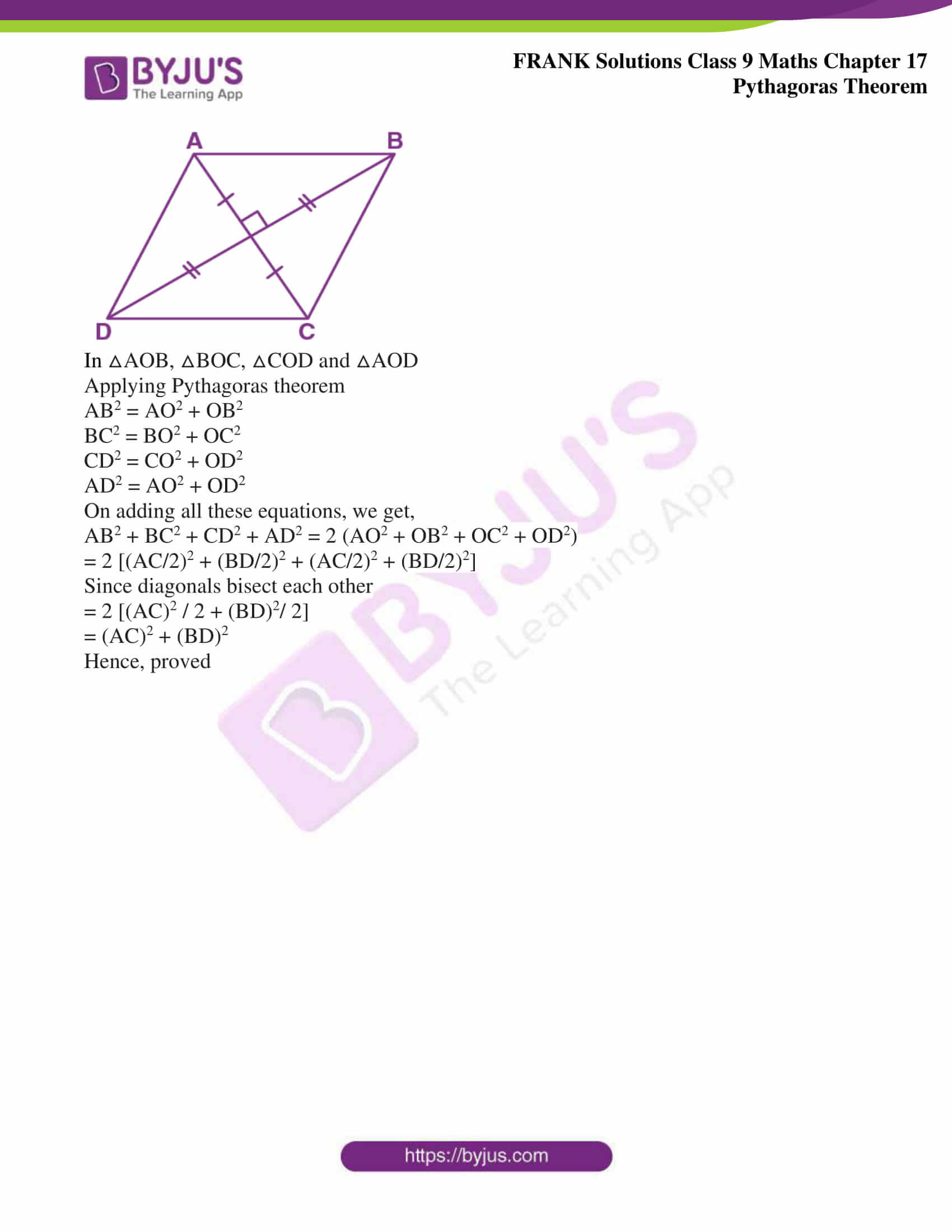## Access Frank Solutions for Class 9 Maths Chapter 17 Pythagoras Theorem

1. Find the length of the perpendicular of a triangle whose base is 5 cm and the hypotenuse is 13 cm. Also, find its area.

Solution:

Given

Base = 5 cm

Hypotenuse = 13 cm

By Pythagoras theorem,

(perpendicular)2 = (13 cm)2 – (5 cm)2

(perpendicular)2 = 169 cm2 – 25 cm2

(perpendicular)2 = 144 cm2

(perpendicular)2 = (12 cm)2

Hence,

perpendicular = 12 cm

Now,

Area of triangle = (1/2) x (Base x Perpendicular)

= (1/2) x 5 x 12

= 5 x 6

We get,

= 30 cm2

2. Find the length of the hypotenuse of the triangle whose other two sides are 24 cm and 7 cm.

Solution:

Given

The two sides (excluding hypotenuse) of a right angled triangle are 24 cm and 7 cm

(hypotenuse)2 = (24 cm)2 + (7 cm)2

(hypotenuse)2 = 576 cm2 + 49 cm2

(hypotenuse)2 = 625 cm2

(hypotenuse)2 = (25 cm)2

(hypotenuse) = 25 cm

Therefore, the length of the hypotenuse of the triangle is 25 cm

3. Calculate the area of a right – angled triangle whose hypotenuse is 65 cm and one side is 16 cm

Solution:

Given

Hypotenuse = 65 cm

One side = 16 cm

Let the length of the other side = x cm

By Pythagoras theorem,

(65 cm)2 = (16 cm)2 + (x cm)2

(x cm)2 = (65 cm)2 – (16 cm)2

(x cm)2 = 4225 cm2 – 256 cm2

On further calculation, we get,

(x cm)2 = 3969 cm2

We get,

(x cm)2 = (63 cm)2

Hence,

x = 63 cm

Area of the triangle = (1/2) x (Base x Height)

= (1/2) x 16 cm x 63 cm

= 8 cm x 63 cm

We get,

= 504 cm2

4. A man goes 10 m due east and then 24 m due north. Find the distance from the starting point.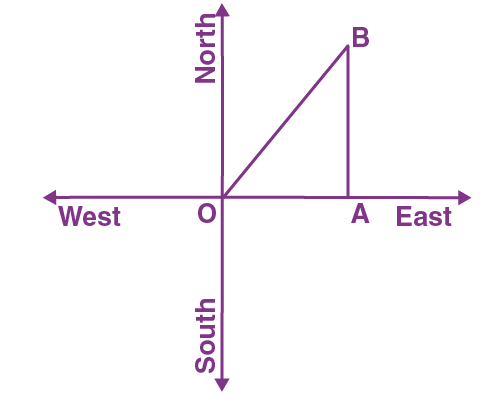Solution:

Let us take ‘O’ as the original position of the man

From the figure, we come to know that B is the final position of the man

Here,

△AOB is right angled at A

By Pythagoras theorem,

OB2 = OA2 + AB2

OB2 = (10m)2 + (24 m)2

OB2 = 100 m2 + 576 m2

OB2 = 676 m2

OB2 = (26 m)2

We get,

OB = 26 m

Therefore, the man is at a distance of 26 m from the starting point

5. A ladder 25 m long reaches a window of a building 20 m above the ground. Determine the distance of the foot of the ladder from the building.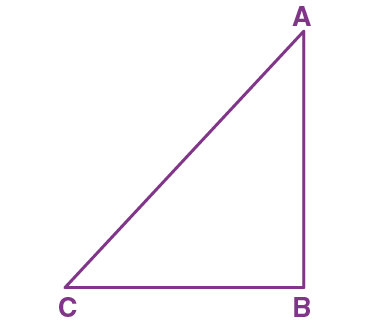Solution:

Let AC be the ladder and A be the position of the window

Then,

AC = 25 m and AB = 20 m

Using Pythagoras theorem,

AC2 = AB2 + BC2

By substituting AC = 25 and AB = 20

We get,

(25 m)2 = (20 m)2 + BC2

BC2 = (25 m)2 – (20 m)2

BC2 = 625 m2 – 400 m2

BC2 = 225 m2

BC2 = (15 m)2

We get,

BC = 15 m

Hence,

The distance of the foot of the ladder from the building is 15 m

6. A right triangle has hypotenuse p cm and one side q cm. If p – q = 1, find the length of the third side of the triangle.

Solution:

Given

Hypotenuse = p cm

One side = q cm

Let the length of the third side = x cm

Using Pythagoras theorem,

x2 = p2 – q2

x2 = (p + q) (p – q)

Substituting (p – q) = 1

We get,

x2 = (p + q) (1)

x2 = (p + q)

x = √p + q

Therefore, the length of the third side of the triangle is √p + q cm

7. A ladder 15 m long reaches a window which is 9 m above the ground on one side of a street. Keeping its foot at the same point, the ladder is turned to other side of the street to reach a window 12 m high. Find the width of the street.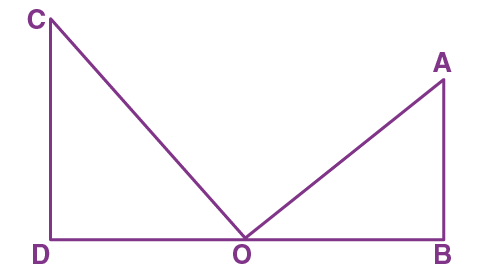Solution:

Let ‘O’ be the foot of the ladder

Let AO be the position of the ladder when it touches the window at A which is 9 m high and CO be the position of the ladder when it touches the window at C which is 12 m high.

Using Pythagoras theorem,

In △AOB,

BO2 = AO2 – AB2

BO2 = (15 m)2 – (9 m)2

BO2 = 225 m2 – 81 m2

On simplification, we get,

BO2 = 144 m2

BO2 = (12 m)2

We get,

BO = 12 m

Using Pythagoras theorem,

In △COD,

DO2 = CO2 – CD2

DO2 = (15 m)2 – (12 m)2

DO2 = 225 m2 – 144 m2

We get,

DO2 = 81 m2

DO = 9 m

Width of the street = DO + BO

= 9 m + 12 m

= 21 m

Therefore, the width of the street is 21 m

8. The foot of a ladder is 6 m away from a wall and its top reaches a window 8 m above the ground. If the ladder is shifted in such a way that its foot is 8 m away from the wall to what height does its top reach?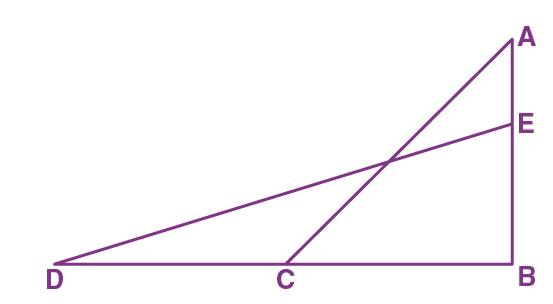Solution:

Let AC be the ladder and A be the position of the window which is 8 m above the ground

Now,

The ladder is shifted such that its foot is at point D which is 8 m away from the wall

Hence,

BD = 8 m

At this instance, the position of the ladder is DE

Hence,

AC = DE

Using Pythagoras theorem,

In △ABC,

AC2 = AB2 + BC2

AC2 = (8 m)2 + (6 m)2

AC2 = 64 m2 + 36 m2

AC2 = 100 m2

We get,

AC = 10 m

We know that, AC = DE = 10 m

Using Pythagoras theorem,

In △DBE,

BE2 = DE2 – BD2

BE2 = (10 m)2 – (8 m)2

BE2 = 100 m2 – 64 m2

BE2 = 36 m2

We get,

BE = 6 m

Hence,

The required height up to which the ladder reaches is 6 m above the ground

9. Two poles of height 9m and 14 m stand on a plane ground. If the distance between their feet is 12m, find the distance between their tops.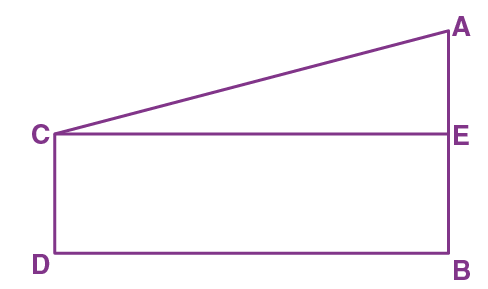Solution:

Let AB and CD be the two poles of 9 m and 14 m respectively

Given that,

BD = 12 m

Thus,

CE = 12 m

Now,

AE = AB – EB

AE = 14 m – 9 m

We get,

AE = 5 m

Using Pythagoras theorem in △ACE,

AC2 = AE2 + CE2

AC2 = (5m)2 + (12 m)2

AC2 = 25 m2 + 144 m2

AC2 = 169 m2

AC2 = 13 m2

We get,

AC = 13 m

Therefore, the distance between the tops of their poles is 13 m

10. The length of the diagonals of rhombus are 24 cm and 10 cm. Find each side of the rhombus.

Solution:

Given

The length of the diagonals of rhombus are 24 cm and 10 cm respectively

Therefore,

d1 = 24 cm and d2 = 10 cm

The diagonals of a rhombus bisect each other

Hence,

(d1 / 2)2 + (d2 / 2)2 = side2

side2 = 122 + 52

side2 = 144 + 25

side2 = 169

side2 = 132

We get,

side = 13

Therefore, each side of the rhombus is of length 13 cm

11. Each side of rhombus is 10 cm. If one of its diagonals is 16 cm, find the length of the other diagonal.

Solution:

Given

Side of the rhombus = 10 cm

Length of one diagonal, d1 = 16 cm

Let d2 be the other diagonal of the rhombus

The diagonals of a rhombus bisect each other

Therefore,

(d1 / 2)2 + (d2 / 2)2 = side2

On further calculation, we get,

82 + (d2 / 2)2 = 102

(d2 / 2)2 = 102 – 82

(d2 / 2)2 = 100 – 64

We get,

(d2 / 2)2 = 62

(d2 / 2) = 6

d2 = 6 x 2

d2 = 12

Therefore, the length of the other diagonal of rhombus is 12 cm

12. In △ABC, AD is perpendicular to BC. Prove that

AB2 + CD2 = AC2 + BD2

Solution: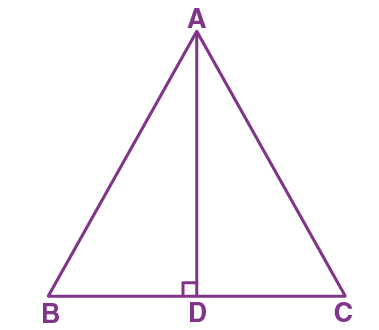Since triangles ABD and ACD are right triangles right angled at D,

AB2 = AD2 + BD2 ……….. (i)

AC2 = AD2 + CD2 ……….. (ii)

Subtracting (ii) from (i), we get,

AB2 – AC2 = BD2 – CD2

We get,

AB2 + CD2 = AC2 + BD2

Hence, proved

13. From a point O in the interior of a △ABC, perpendicular OD, OE and OF are drawn to the sides BC, CA and AB respectively. Prove that:

(a) AF2 + BD2 + CE2 = OA2 + OB2 + OC2 – OD2 – OE2 – OF2

(b) AF2 + BD2 + CE2 = AE2 + CD2 + BF2

Solution: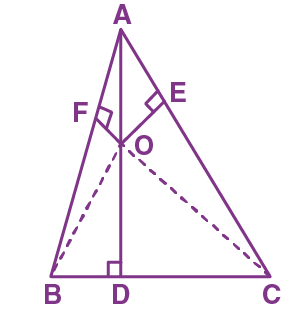(a) In right triangles OFA, ODB and OEC,

We have,

OA2 = AF2 + OF2

OB2 = BD2 + OD2

OC2 = CE2 + OE2

By adding all these results, we get,

OA2 + OB2 + OC2 = AF2 + BD2 + CE2 + OF2 + OD2 + OE2

AF2 + BD2 + CE2 = OA2 + OB2 + OC2 – OD2 – OE2 – OF2

Hence, proved

(b) In right triangles ODB and ODC,

We have,

OB2 = OD2 + BD2

OC2 = OD2 + CD2

On subtracting, we get,

OB2 – OC2 = (OD2 + BD2) – (OD2 + CD2)

We get,

OB2 – OC2 = BD2 – CD2 …….(1)

Similarly, we have,

OC2 – OA2 = CE2 – AE2 ……..(2)

OA2 – OB2 = AF2 – BF2 …….. (3)

Adding equations (1), (2) and (3) we get,

(OB2 – OC2) + (OC2 – OA2) + (OA2 – OB2) = (BD2 – CD2) + (CE2 – AE2) + (AF2 – BF2)

On further calculation, we get,

(BD2 + CE2 + AF2) – (AE2 + CD2 + BF2) = 0

AF2 + BD2 + CE2 = AE2 + CD2 + BF2

Hence, proved

14. A point O in the interior of a rectangle ABCD is joined with each of the vertices A, B, C and D. Prove that OB2 + OD2 = OC2 + OA2

Solution:

Let ABCD is the given rectangle and ‘O’ be a point within it

Join OA, OB, OC and OD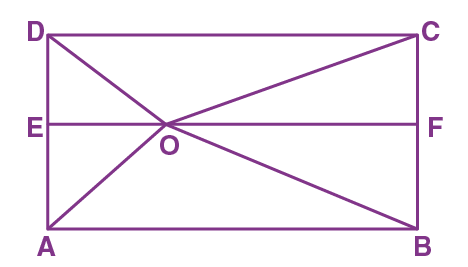Through ‘O’, draw EOF || AB

Then, ABFE is a rectangle

In right triangles △OEA and △OFC,

We have,

OA2 = OE2 + AE2 and

OC2 = OF2 + CF2

OA2 + OC2 = (OE2 + AE2) + (OF2 + CF2)

OA2 + OC2 = OE2 + OF2 + AE2 + CF2 …… (1)

Now,

In right triangles △OFB and △ODE,

We have,

OB2 = OF2 + FB2 and

OD2 = OE2 + DE2

OB2 + OD2 = (OF2 + FB2) + (OE2 + DE2)

OB2 + OD2 = OE2 + OF2 + DE2 + BF2

OB2 + OD2 = OE2 + OF2 + CF2 + AE2 …….(2)

∵ DE = CF and AE = BF

From equations (1) and (2) we get,

OA2 + OC2 = OB2 + OD2

Hence, proved

15. ABCD is a rhombus. Prove that AB2 + BC2 + CD2 + DA2 = AC2 + BD2

Solution: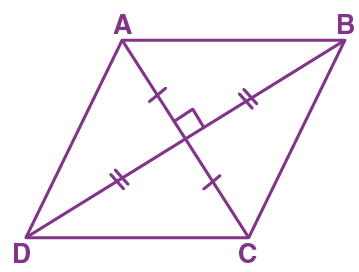In △AOB, △BOC, △COD and △AOD

Applying Pythagoras theorem

AB2 = AO2 + OB2

BC2 = BO2 + OC2

CD2 = CO2 + OD2

On adding all these equations, we get,

AB2 + BC2 + CD2 + AD2 = 2 (AO2 + OB2 + OC2 + OD2)

= 2 [(AC/2)2 + (BD/2)2 + (AC/2)2 + (BD/2)2]

Since diagonals bisect each other

= 2 [(AC)2 / 2 + (BD)2/ 2]

= (AC)2 + (BD)2

Hence, proved